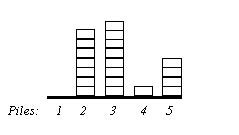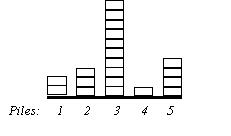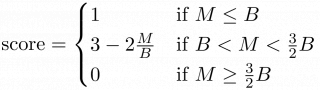## Flatten

A solitaire game is played given a row of N piles where each pile contains zero or more chips. See Figure 1. Piles are identified by integers 1 through N. In a move of this game you point to a pile, say p, and specify a number, say m. Then m chips are transferred from the pile p to each of its neighboring piles. See Figure 2 for an example. Pile p has two neighbors, p-1 and p+1 if 1<p<N, and the neighbor 2 if p=1, and the neighbor N-1 if p=N. Note that to be able to make such a move the pile p must have at least 2m chips if it has two neighbors, and it must have at least m chips if it has only one neighbor.

The object of the game is to "flatten" all piles, that is make them have the same number of chips, by making as small as possible number of moves. In case there is more than one solution you are required to report only one of them.Figure 1. Five piles with 0, 7, 8, 1 and 4 chips. Figure 2. Configuration of same piles after a move: p=2, m=2.

### Input

The first line contains the integer N (2 ≤ N ≤ 200).
The second line contains N integers, C1, C2, ... CN, where Ci is the number of chips in the ith pile (1 ≤ Ci ≤ 2000 at the start of the game, but not necessarily later on.)
It is guaranteed that it is possible to flatten the given piles, and that doing so will require no more than 10000 moves.

### Output

The first line should contain the number of moves M used by your solution.
Each of the following M lines should describe one move as two space-separated integers p and m. p should be the id number of the pile and m the number of chips transferred to each adjacent pile (not necessarily the total number of chips transferred.) Of course, the moves should be in the correct order in the output file.

### Sample Input

5
0 7 8 1 4


### Sample Output

5
5 2
3 4
2 4
3 1
4 2


### Scoring

The judges have set a target number of moves B for each test case. If your program supplies a correct solution that uses M moves, your score, a number between 0 and 1, will be determined as follows:$\displaystyle \mathrm{score} = \begin{cases} 1 & \text{if M \le B} \\ 3 - 2\frac{M}{B} & \text{if B < M < \frac{3}{2}B} \\ 0 & \text{if M \ge \frac{3}{2}B } \end{cases}$
If your program supplies an incorrect solution, you will receive a score of zero for that testcase.

Point Value: 30 (partial)
Time Limit: 2.00s
Memory Limit: 16M